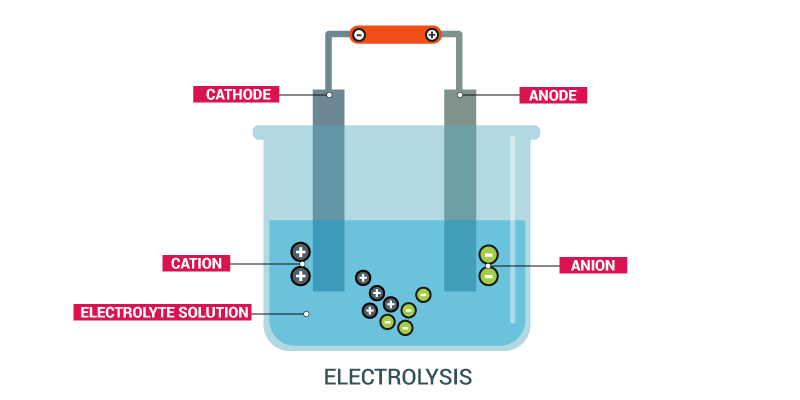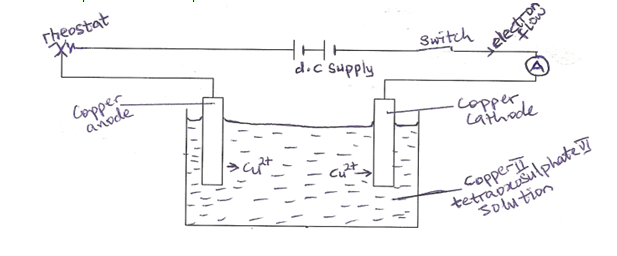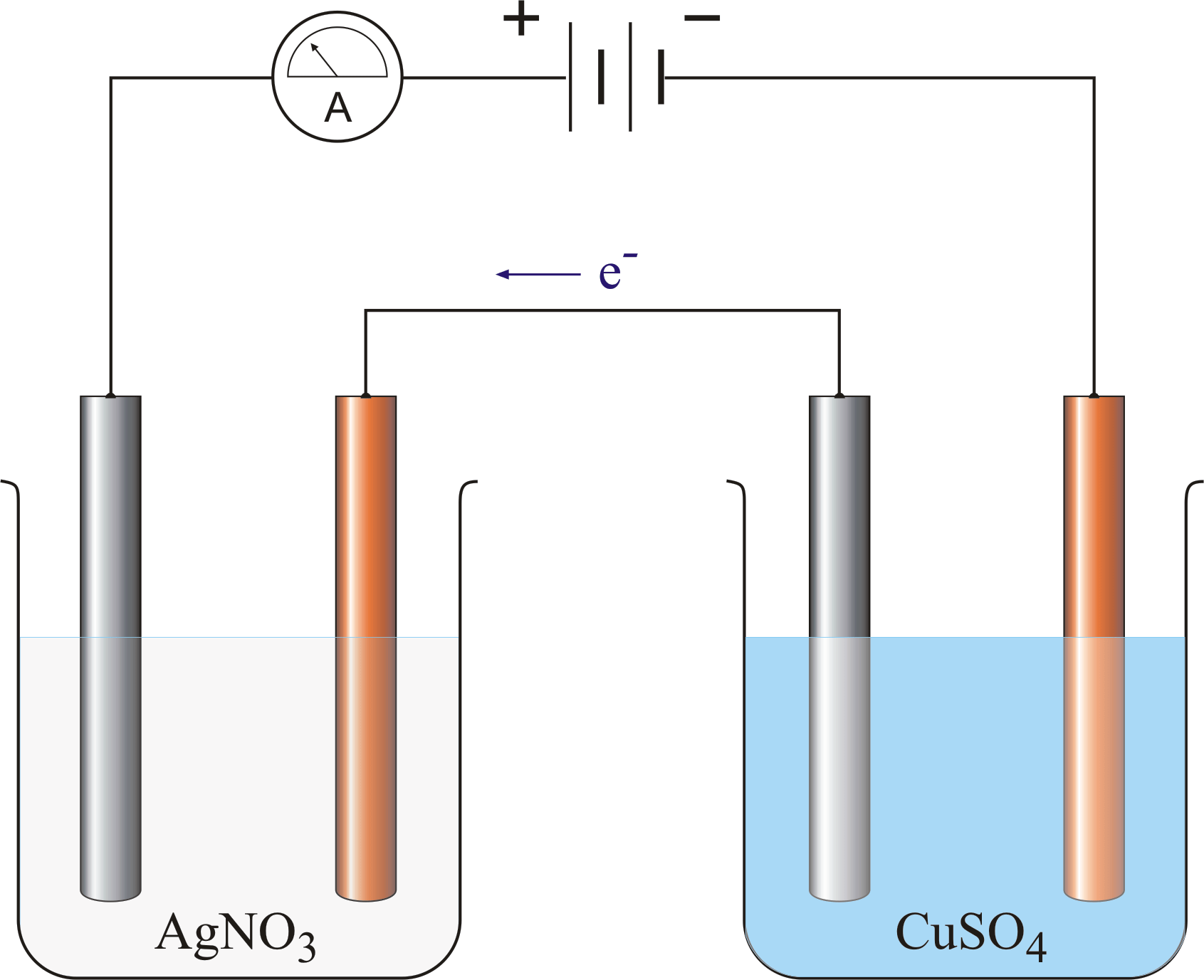Welcome to Class !!

In today’s Chemistry class, We will be learning about Faraday’s Laws of Electrolysis. We hope you enjoy the class!CONTENT

• Faraday First and Second laws of electrolysis.
• Verification of Faraday’s Laws of Electrolysis.
• Calculations based on Faraday’s Laws.

#### FARADAY’S FIRST LAW OF ELECTROLYSIS

Faraday’s first law states that the mass (m) of an element discharged during the electrolysis of an electrolyte is directly proportional to the quantity of electricity (Q) passing through it.

Faraday’s first law can be expressed in the following mathematical way

M α Q

But Q = It

M α It

M =EIt   or                 M = EQ

Where E is the constant of proportionality known as Electrochemical equivalent of a substance.

M is measured in gram (g)

I is measured in Amperes (A)

t is measured in seconds (s)

Q is measured in Coulomb(C)

Therefore E is measured in gA-1S-1 or gC-1, for a particular electrolytic process at different time, the law can also be expressed as M1/Q1 = M2/ Q2

OR :-   M1       =          M2

I1 t1                  I2 t2

VERIFICATION OF FARADAY’S FIRST LAW OF ELECTROLYSIS.

The experimental set up isThe electrolytic cell above can be used to verify Faraday’s first law.

The copper cathode is weighed at the beginning of the experiment. A steady current of 1A is passed for 10mins and the current supply switched off. The copper cathode is then weighed again to find the gain in mass. This procedure is repeated using different time intervals. A graph of gain in mass against time gives a straight line passing through the origin.

The copper cathode is then weighed again to find the gain in mass. This procedure is repeated using different time intervals. A graph of gain in mass against time gives a straight line passing through the origin.

When the experiment is repeated using different currents for a fixed period of time, a graph of gain in mass against the magnitude of the current also gives a straight line passing through the origin.The two graphs show that the mass of copper deposited is directly proportional to the magnitude of the current, i.e M α I

EVALUATION

1. State Faraday’s first law of electrolysis and write a mathematical expression for the law
2. What kinds of graphs are obtained when mass is plotted against time or mass is plotted against current in electrolysis?

#### FARADAY’S SECOND LAW OF ELECTROLYSIS

Faraday’s second law of electrolysis states that when the same quantity of electricity Q is passed through different electrolytes, the relative number of mole of the elements discharged is inversely proportional to the charges on the ions of the elements.

The minimum quantity of electricity required to liberate one mole of a univalent ion is 96500C. This quantity of electricity is called the Faraday (F). One Faraday (1F) is one mole of electrons which contains Avogadro’s number (6.62 x 1023) of electrons. It follows that two Faraday’s (2F) will be required to discharged 1 mole of ions of a divalent element or radical such as Pb2+ or S042-

i.e M2+    +     2e-                      M         or          A2-                       A    +      2e-

VERIFICATION OF FARADAY’S SECOND LAW OF ELECTROLYSIS

To verify the second law, set up two electrolytic cells.

i.     One with copper electrodes immersed in CuSO4 solution

ii     The other with silver electrodes immersed in AgNO3 solution.

The copper and silver cathodes are weighed before the experiment begins

A steady current of 1A is passed into the solution say for 15mins and the current supply switched off. The mass of each cathode is then weighed again to find the gain in mass. The number of moles of silver deposited at the silver cathode will be doubled the number of copper deposited at the copper cathode although the same quantity of electricity was passed through the two electrolytes i.e. if Y moles of copper are deposited by 2Y moles of silver are deposited. The charge on the Cu2+ is +2 and that on the Ag+ is +1 hence, the number of moles of copper and silver deposited is inversely proportional to charge on their ions###### CALCULATIONS BASED ON THE LAW

EXAMPLES

1.    Calculate the electrochemical equivalent of a metal M if a current of 5A is passed for 1hr and 2g of M is deposited

SOLUTION:

:-          M = 2g,          I = 5A,            t = 1hr

M = Z It

2 =  Z x 5x 1x 60x 60            = 2/1800

Z = 2.78 x 10-4 g I-1 s-1

2     During electrolysis of a copper solution 5g of copper was deposited in 10min when a current of 1A was passed. Calculate the time required to deposit 20g

• A. if the same current was passed
• B. if a current of 2A was passed.

SOLUTION:-

M1, = 5g, t1 = 10min = 600sec.

I1 = 1A

A. I1 = I2 = 1A,                M2 = 200g, t2= ?

B.   I2 = 2A , M2 = 20g, t= ?

EVALUATION

1. What mass of aluminium will be deposited if 5.6A is passed through its solution for 10minutes?
2. Calculate the mass of silver deposited when a current of 2.6A is passed through a solution of a silver salt for 70 minutes. (Ag = 108; 1 Faraday = 96500C)

WORKED EXAMPLES

1. How many moles of electrons are required to discharge 2.5 moles of silver ion during electrolysis?

SOLUTION:

Ag++  e                  Ag

1 Mole Ag+ is discharged by 1-mole e

2.5 moles Ag+ are discharged by 2.5-mole e

2.5 moles of electrons are required

1. If 6 moles of electrons are passed through different solutions of copper and silver, calculate the moles of silver deposited by the time 3 moles of copper have been deposited

SOLUTION

Cu2+     +   2e                                   Cu

1 mole of Cu is deposited by 2 moles of e

3 moles Cu is deposited by 6 moles of e

For silver:

Ag+    +   e                     Ag

1 mole of edeposits 1 moles of Ag

6 moles of ewill deposit 6 moles of Ag

### APPLICATIONS OF ELECTROLYSIS

Electrolysis is applied in the industries in the following areas e.g.

1. Extraction of elements: Na, K, Mg, Ca, Al, Zn and nonmetal e.g. H2, F2, Cl2 are obtained either by electrolysis of their ores or by the electrolysis of their fused compounds or their aqueous solutions.
2. Purification of metals e.g. Cu, Hg, Ag, Au
3. Electroplating of one metal by another
4. Preparation of certain important compounds such as sodium hydroxide, and sodium trioxochlorate (V)

#### EXTRACTION AND PURIFICATION

Very electropositive metals e.g. Ca, Mg, and Al and electronegative non-metal e.g. F and Cl are extracted from their compounds by electrolysis.

The less electropositive metals e.g. copper, mercury, silver and gold occur in the uncombined ores mixed with impurities. The pure metals are obtained from the ore by electrolytic purification.

During electrolytic purification of copper ores, the impure copper is made the anode while a pure copper is made the cathode. The electrolyte is usually copper (II) tetraoxosulphate(VI) solution.

At the anode:- The metallic copper atoms in the ore give up two electrons each forming copper II ions and move into the solution.

Cu(s)                          Cu2+(aq)   +      2e

Electrolytic Purification of Copper#### ELECTROPLATING

Electroplating is a method of coating the surface of one metal with another metal, usually copper, silver, chromium, nickel, or gold by means of electrolysis. The main objectives of electroplating are

1. Decoration
2. Protection against corrosion.

Articles which are usually plated include cutlery, jewellery and iron or steel objects such as the metallic parts of motor carsWEEKEND ASSIGNMENT

SECTION A: Write the correct option ONLY

1          In silver plating a spoon, the spoon must be made the

A. Anode           B.        Cathode      C.         Electrode      D.        Electrolyte

2          A current of 20A is passed for 30 minutes during the electrolysis of gold (III) tetraoxosulphate (VI). The mass of gold deposited at the cathode is

A. 24.4g      B.        26.5g    C.   28.9g  D.        32.6g

3          What quantity of chlorine in moles is liberated when a current of 15A is passed through molten potassium chloride in 1930 seconds?

A. 0.05                B.        0.10                C.         0.15                D.        0.20

4          What quantity of silver is deposited when 96500C of electricity is passed through a solution containing silver ions [Ag = 108, F = 96500C]

A. 1.08g              B.        10.8g              C.         108g               D.        5.40g

5          Calculate the volume of oxygen liberated at s.t.p. when 96500C of electricity is passed through an aqueous solution of H2S04. [IF = 96500C, Volume at s. t. p = 22.4 dm3]

A. 5.6dm3    B.        0.56dm3        C.         56dm3            D.        560dm3

SECTION B

1. In the electrolysis of dilute CuSO4 solution using platinum electrodes, 2.0A current passed for 2.50hrs and deposited 5.85g of copper
2. Calculate the relative atomic mass of copper from the experiments
3. What volume of gas is liberated at s.t.p.?
4. Using a well-labelled diagram only, explain the process of silver plating a spoon.
5. Give three uses of electrolysis

We have come to the end of this class. We do hope you enjoyed the class?

Should you have any further question, feel free to ask in the comment section below and trust us to respond as soon as possible.

In our next class, we will be learning about Electrochemical Cells. We are very much eager to meet you there.

Are you a Parent? Share your quick opinion and win free 2-month Premium Subscription

Don`t copy text!